# Bonds vs Stocks

The former is also referred to as fixed-income securities, while the latter is often addressed as equity.

Bonds and stocks are two asset classes that are commonly included in diversified portfolios. Bonds are also fixed-income securities, while stocks are often addressed as equity.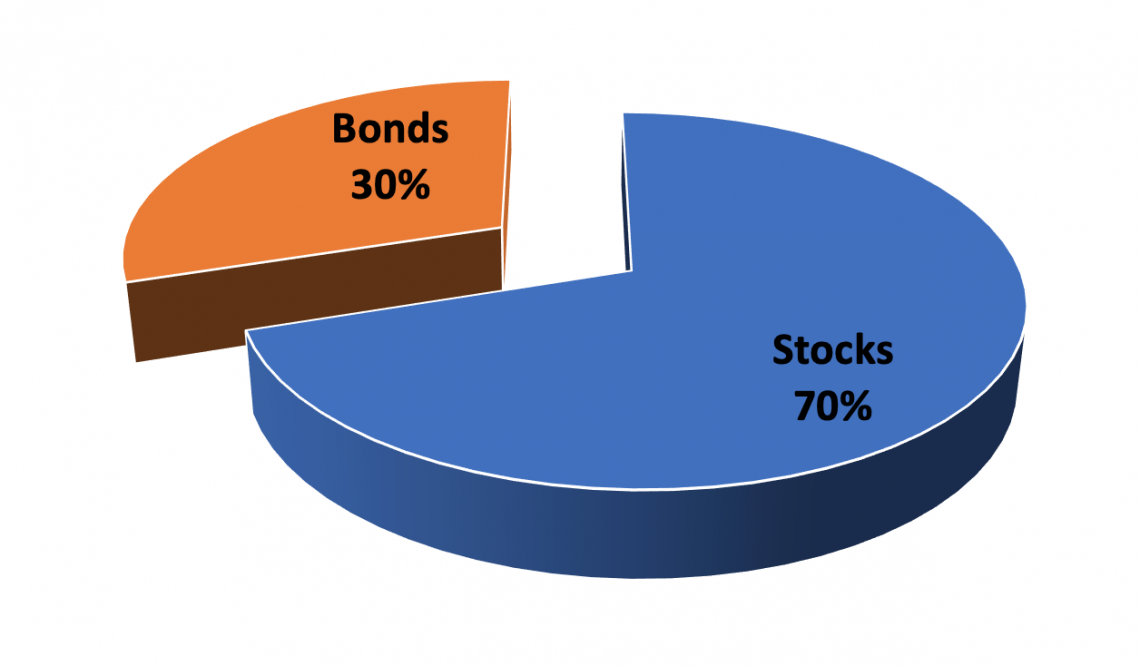Understanding bonds, stocks, and differences are important for companies and investors.

From a corporate perspective, financing by issuing stocks is:

• More expensive than issuing bonds
• Less risky than issuing bonds

From an investor perspective, purchasing stocks:

• Can generate higher rates of return than buying bonds
• Is riskier than investing in bonds

A simple way to understand the company-investor relationship in the financial markets is in terms of risk.

Stocks are riskier than bonds, so they must offer investors higher returns. However, because these are safer alternatives for investors, financing with bonds is a cheaper alternative for companies.

## Bonds

Bonds, or fixed income securities, serve as a debt contract between borrowers and lenders where payments are made on a set schedule, allowing lenders to loan money to borrowers in return for a schedule of fixed payments in the future.

The origins of the bond market come from government budget deficits when a government's expenditure is greater than its income from taxes.

Purchasers of US government bonds, for example, are financing the national debt with the expectation of future payments from the US government.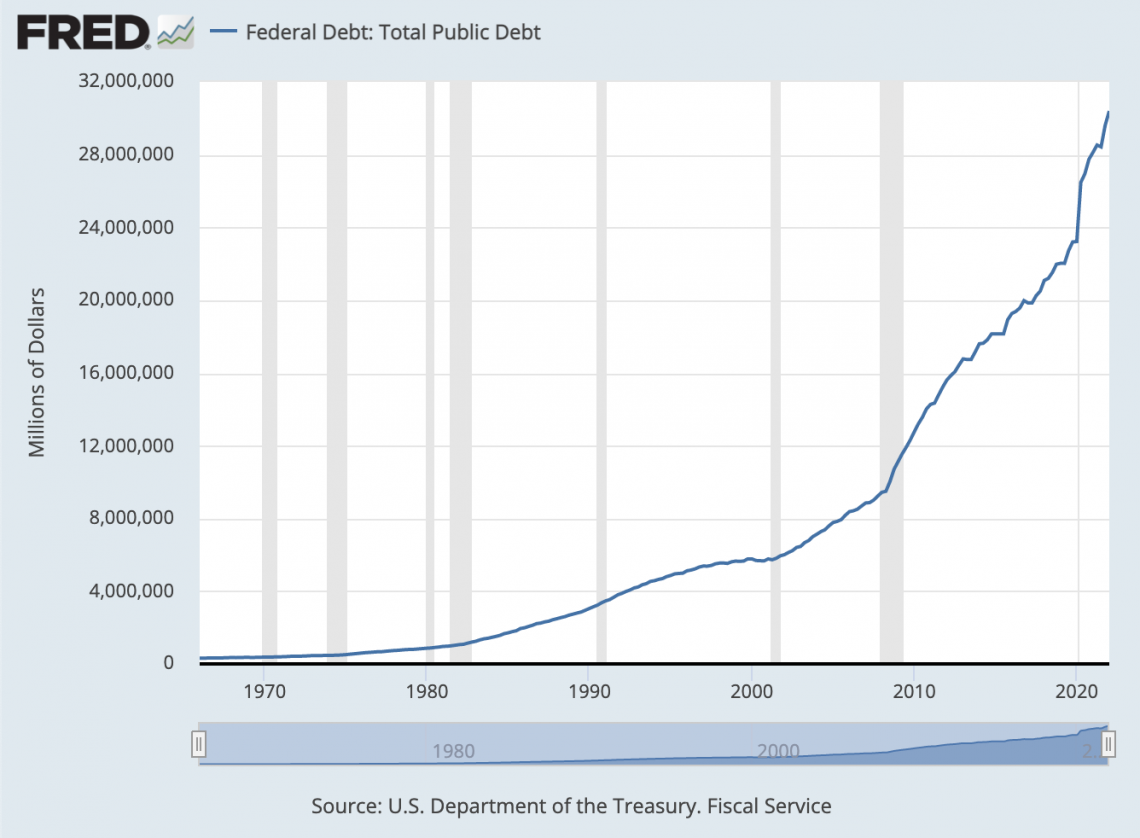These fixed payments are coupons that the issuer of the bond pays back at a rate defined in the contract's original terms.

When a bond reaches maturity, meaning the end of the coupon payment period, the principal, which is the amount of debt financed when the bond is issued, is also repaid.

The principal, the maturity, and the coupon rate are determined before a bond is issued. After a bond is issued, it is often traded back and forth on the secondary market.

In addition to government bonds, corporations sell bonds to take advantage of debt financing. Selling corporate bonds is advantageous compared to other forms of financing for a few reasons.

First, repaying debt lowers taxable income because debt is counted as an interest expense on the income statement before tax. This tax benefit lowers the cost of capital

In addition, because of the fixed repayment schedule, debt is inherently less risky for investors than equity, which does not necessarily guarantee dividend payments. As a result, the investor's required rate of return from the issuing company is lower for debt compared to equity.

Although debt is cheaper for corporations, it is also riskier than issuing equity as there is a fixed repayment schedule, a liability that reduces their profits.

The decision to sell bonds or raise money by issuing equity is complex. A corporation's mix of financing is known as its capital structure

## Bond Valuation

To calculate the value of a bond, we need to understand the concept of the time value of money. Deciding between having \$100 now versus \$100 in ten years is quite simple. The money is certain, but there is no telling what will happen ten years from now.Besides the uncertainty of tomorrow, inflation and interest rates also reduce the present value of money received in the future.

A 2% annual inflation rate would mean that money will have 2% less purchasing power each year. This is important because consumers can afford less with the same amount of money.

In addition, interest rates reduce the present value of money, as the present value of money is discounted using relevant interest rates.

If you decide to have \$100 today, its present value is \$100. In contrast, if you chose to receive the \$100 in ten years, the present value of that \$100 in the future would not equal \$100 now.

To find the present value of that \$100 in ten years, we need to think back and figure out how much money we need to put in a bank account today for it to grow into a \$100 value in ten years.

Hence, having money now is more attractive than in the future because investors can earn interest by putting the money into a bank account. In contrast, if they choose to receive money in the future, they forgo the interest they can earn.

Let us quantify the above statement using another example: assuming that we have \$100 in the present and put it in a bank account that earns interest of 3% annually.

We can calculate the future value of that money after five years with the following equation, assuming that we can reinvest earned interest:

Future Value = 100(1 + .03)5

In this example, \$100 is the present value of our money, .03 is the annual rate of return, and 5 is the number of years into the future we are looking for. More generally, we can express this relationship in the following way:

Future Value = Present Value(1 + r)n

Rearranging this relationship, we can derive a formula for the present value of money.

Present Value = Future Value/(1 + r)n

From this formula, we can see the inverse relationship between the present value and the interest rate. When the interest rate is higher (because it is in the denominator), the present value is lower, and vice versa.

Looking back at the bond example, we can now find the present value of a bond. We know that a bond offers a series of fixed payments over time. So the bond's present value is the sum of the present value of all of these future payments.

Now we can understand where a bond's price comes from. A bond's price is how much investors will pay to lock in those future payments. Typically, it is the present value of those payments.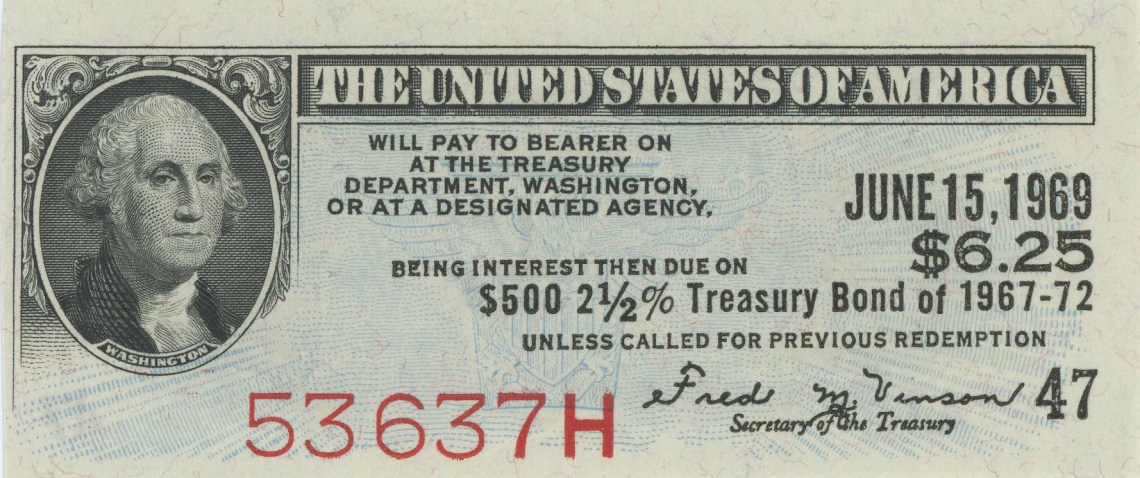If the bond price was higher than the value of those payments, people would not buy, which would lower the price. But conversely, if the price were lower than the value of those payments, the price would rise with the increase in demand.

Bond investors are primarily interested in a bond's yield or its annual rate of return. However, looking at the formula for present value, we can understand that the yield is inversely related to price. Therefore, let us modify our formula to encapsulate the many coupon payments in a bond.

The formula gives the present value: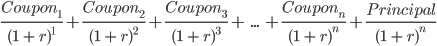In a bond contract, the coupon payments and principal are specified. The present value is the price. This means that if we solve for r, we can find the bond's yield to maturity.

A simple way of thinking about a bond's yield is the equivalent interest rate on a bank account that would provide an identical payment over the loan's lifespan, assuming interest payments are reinvested.

Although we work backward from the price to determine a bond's yield above, investors' required yield sets the bond's price in real life. There are a couple of factors that influence this:

• Default risk
• Maturity risk

We define the required rate of return as the sum of the risk-free rate and any risk premiums. Corporate bonds generally have a higher default risk than government bonds, which are assumed never to default.

Because of this extra risk, investors need greater compensation, or they would not invest, as there is a less risky alternative with the same return.

The difference in yield between bonds with the same maturity but different credit quality is called the credit spread. It measures the riskiness of a corporate bond against a risk-free government bond.In addition to the default risk, a premium is associated with the time until maturity. This is because a longer period until maturity means more time for interest rates to change, impacting the risk-free rate.

Changes in the risk-free rate affect the entire yield curve and can make a bond lose value, as newer bonds offer a higher yield for less risk.

This is because, in situations where the risk-free rate has increased, new bonds will offer higher yields to satisfy investors' demands for higher rates of return. For older bonds offering lower yields, demand would decrease, lowering the price.

To capture the risk of price change from interest rate sensitivity, the maturity risk premium is also included in the price of a bond.

Overall, understanding these risk premiums helps understand the differences in their yields and subsequently compare bonds.

## Stocks

Much like bonds, equities are issued by corporations as a way of raising money. However, unlike bonds, a share of company stock is not a form of debt that ensures a fixed payment. Rather, holding equity in a company gives partial ownership of residual cash flows the company generates.By nature, these cash flows vary from year to year, and they are also not guaranteed. The inherent volatility of returns is why stocks are much riskier of an investment than bonds.

Moreover, in the case of bankruptcy, all liabilities must be paid back before shareholders get anything. These liabilities include bonds. Hence, stockholders are at the bottom of the payment food chain in liquidation.

As discussed in the fixed income section, investors require compensation for the added equity risks. For this reason, equity financing is more expensive than corporate debt financing.

Equity valuation is similar to the valuation of securities trading in the bond market because it is also governed by the concept of the time value of money.

There are two primary ways of determining equity value; these include absolute valuation and relative valuation.

• Absolute Valuation:

Absolute valuation is conducted in a manner almost identical to calculating the present value of a bond. As a company shareholder, you are a partial owner of its future cash flows. The value of your share, or price per share, is therefore directly linked to those future earnings.However, unlike a bond with fixed payments, the future earnings of stock are impossible to know. So instead, equity analysts try to forecast these future earnings by considering past earnings and using various economic indicators.

After forecasting future earnings, we must discount these values, much like calculating the bond price. The discount rate differs for each company, depending on the capital structure of the individual company and its volatility compared to the stock market as a whole.

To determine the discount rate, we look at the weighted average cost of capital, or WACC

WACC = (Weight of Debt) x (Cost of Debt) + (Weight of Equity) x (Cost of Equity)

In this equation, the weight of debt and equity are unique to a company's capital structure. These weights are determined by how a company is financed – the percentage of capital raised from issuing debt compared to equity.

The cost of debt is the yield on bonds issued by the company plus any other debt taken out as a weighted average. This is expressed as an annual percentage.

On the other hand, the cost of equity is a bit more complicated, and analysts like the earnings forecast also estimate it. A simple way to look at the cost of equity is by looking at the Beta of the stock, that is, the volatility of the stock compared to the overall market index.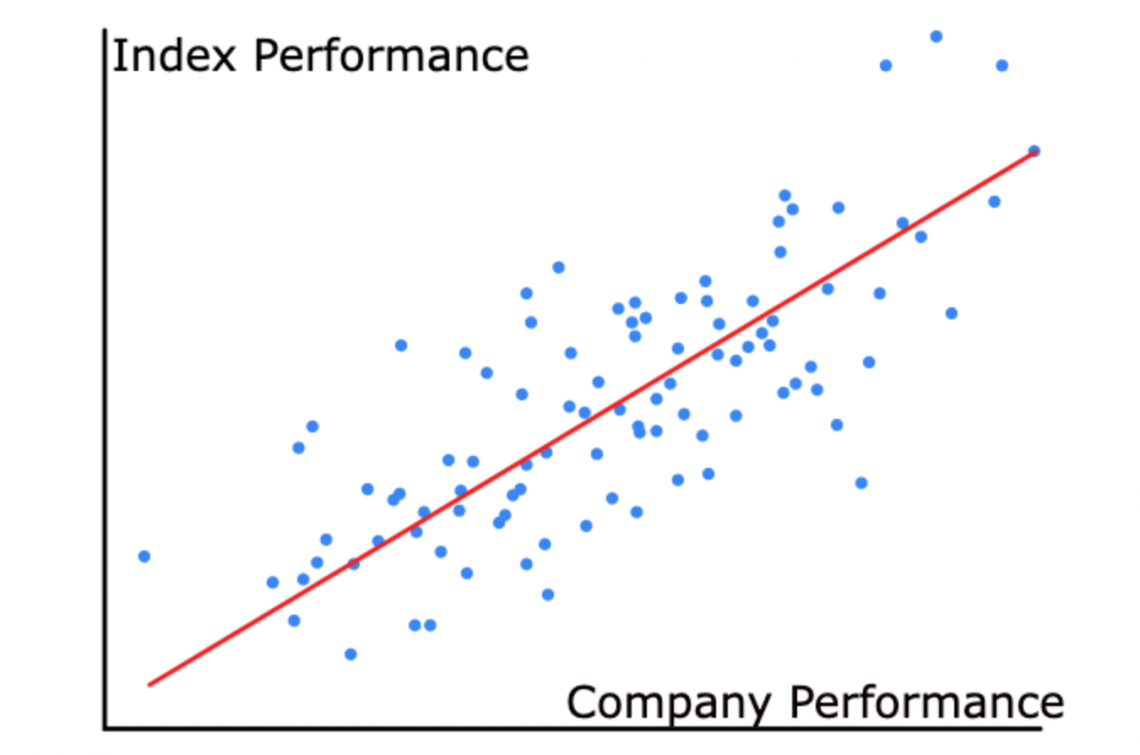The cost of equity equals Beta multiplied by the market risk premium plus the risk-free rate. The market risk premium is the difference between the market and risk-free rates.

Cost of Equity = Risk-Free Rate + β(Market Rate - Risk-Free Rate)

The market rate is the stock market's expected return, while the risk-free rate is the Treasury market bond yield for a specified period. For an investment horizon of 10 years, it would be logical to use the ten-year Treasury bond yield as a risk-free rate.

Last, we discount future cash flows using the WACC, subtract debt, and add cash and equivalents. The remaining value is the company's market capitalization, which can be divided by the number of shares outstanding to derive a fair equity price per share.

• Relative Valuation:

Unlike absolute valuation, which looks to find a fair market value and price for a stock, relative valuation looks at a company's stock in relation to competitors. This can tell us if a stock is undervalued or overpriced and whether it could be a buy.We have established that the fair price of a stock is determined by expectations of the company's future earnings. Therefore, the current price of a stock can be compared to its earnings per share to create the price-to-earnings ratio or P/E ratio.

By comparing respective P/E ratios, we can see how share prices in an industry differ between companies in terms of earnings per share.

A company with a lower P/E ratio than the industry average could be considered undervalued. Conversely, a high P/E ratio means that the stock's price is high in relation to earnings and is possibly overvalued

It is also useful to look at the P/E ratio of a company over time. A rising P/E ratio indicates that investors are more excited about investing in a company than in the past. This can be for some reasons, one of which is the expectation of sharp earnings growth.

Key Takeaways

Stocks and bonds are both issued by companies to raise capital. Investors can invest in stocks and bonds or create a portfolio with a mixture of both.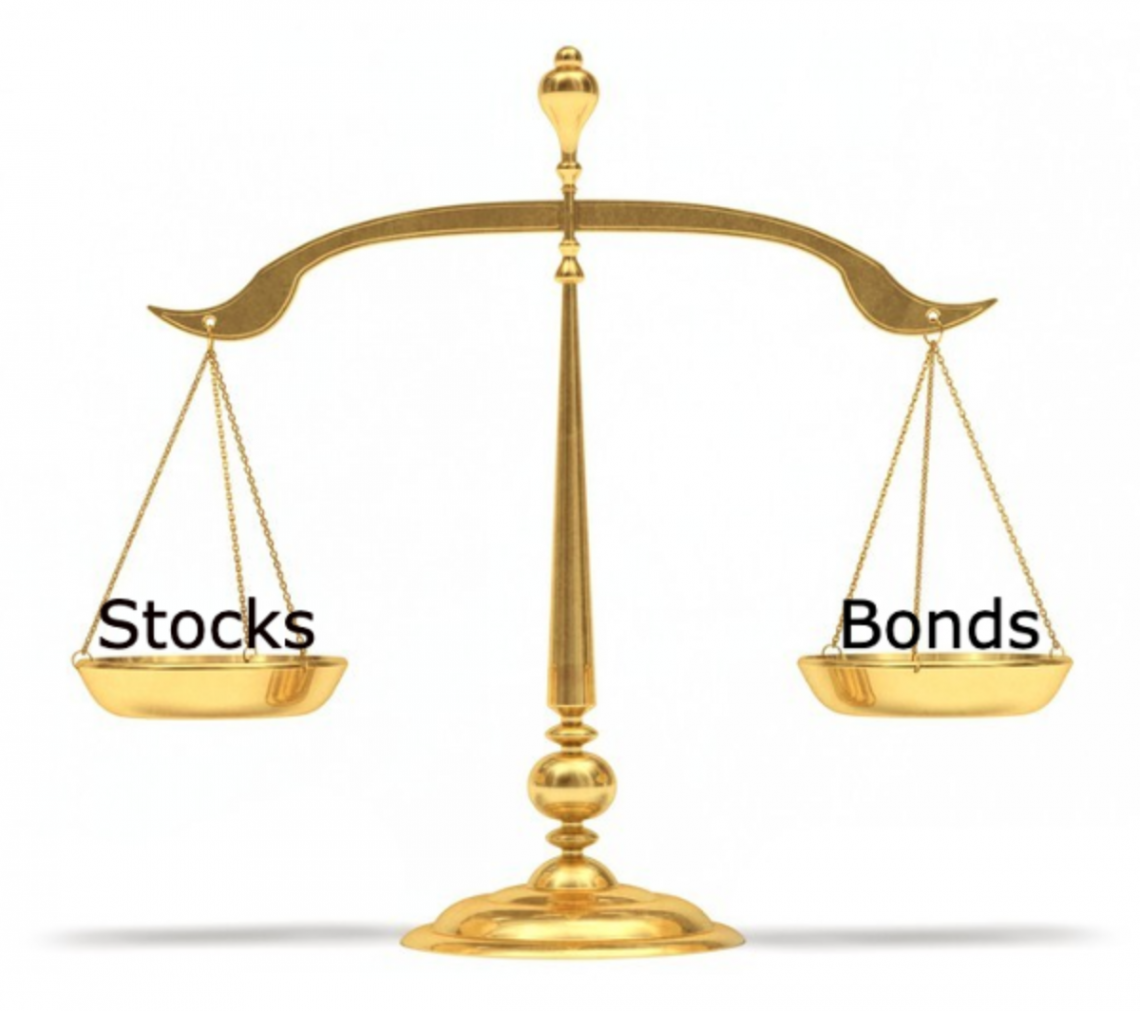1. Bonds are considered less risky for investors because they offer fixed repayments for a set period.

2. Stocks are riskier for investors as there are no fixed payments. Returns in the form of either dividends or capital gains are uncertain.

3. Bonds offer a lower rate of return than stocks for investors because they are less risky.

4. For companies, bonds are less expensive than stocks but riskier.

5. Conversely, stocks are less risky for companies but more expensive to issue than bonds.

6. We can derive the fair value of stocks and bonds by looking at their future payments or our expectations of those payments and discounting them to find their present value.

7. We can compare equity prices using the price-to-earnings (P/E) ratio.

8. We can use bond prices and their payout schedules to compare bond yields.

Both stocks and bonds have different advantages and drawbacks. While there are existing valuation methods, investment decisions are completely up to individual investors' preferences.### Everything You Need To Build Your Accounting Skills

To Help You Thrive in the Most Flexible Job in the World.

Researched and Authored by Jacob Rounds | LinkedIn

Reviewed and Edited by Hongmo Liu | LinkedIn